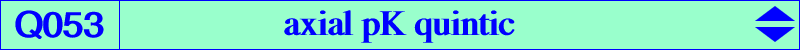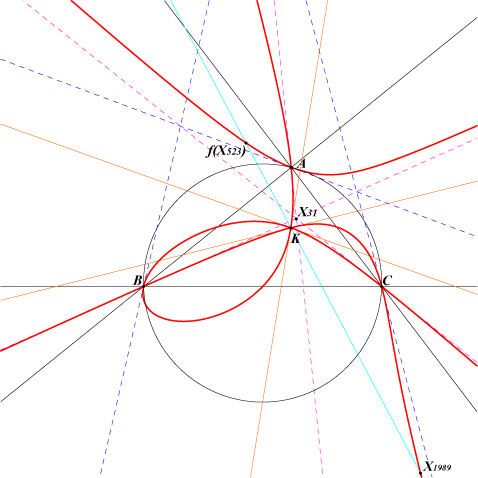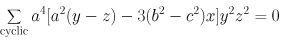X(6), X(1989) midpoints of ABC Ωa, Ωb, Ωc see Table 41 and CL052Q053 is the locus of poles of axial pK isocubics. This means that, for every W on Q053, there are axial pKs with pole W although they might not be always real. See Special Isocubics §3.5.4. Q053 is a quintic passing through K (triple point), A, B, C (double points). The tangents at A, B, C are the cevians and the anticevians of X(31), the second power point. Q053 is the transform of the line at infinity under the mapping f : u:v:w -> (SB v - SC w) / (c^2v^2 - b^2w^2) : : . The infinite points of two perpendicular lines are mapped to two points collinear with K. For example, X(30) and X(523) are mapped to X(1989) and f(X523) not mentioned in the current edition of ETC. See the related K095 and K678.